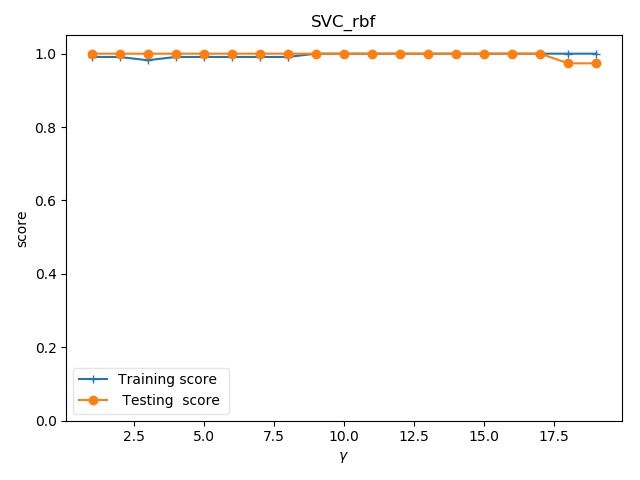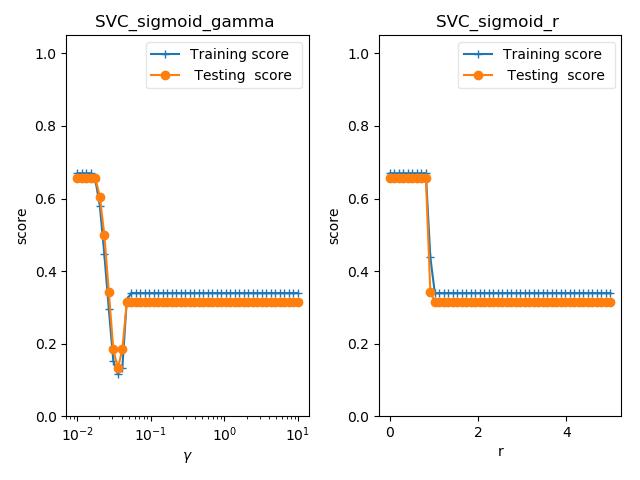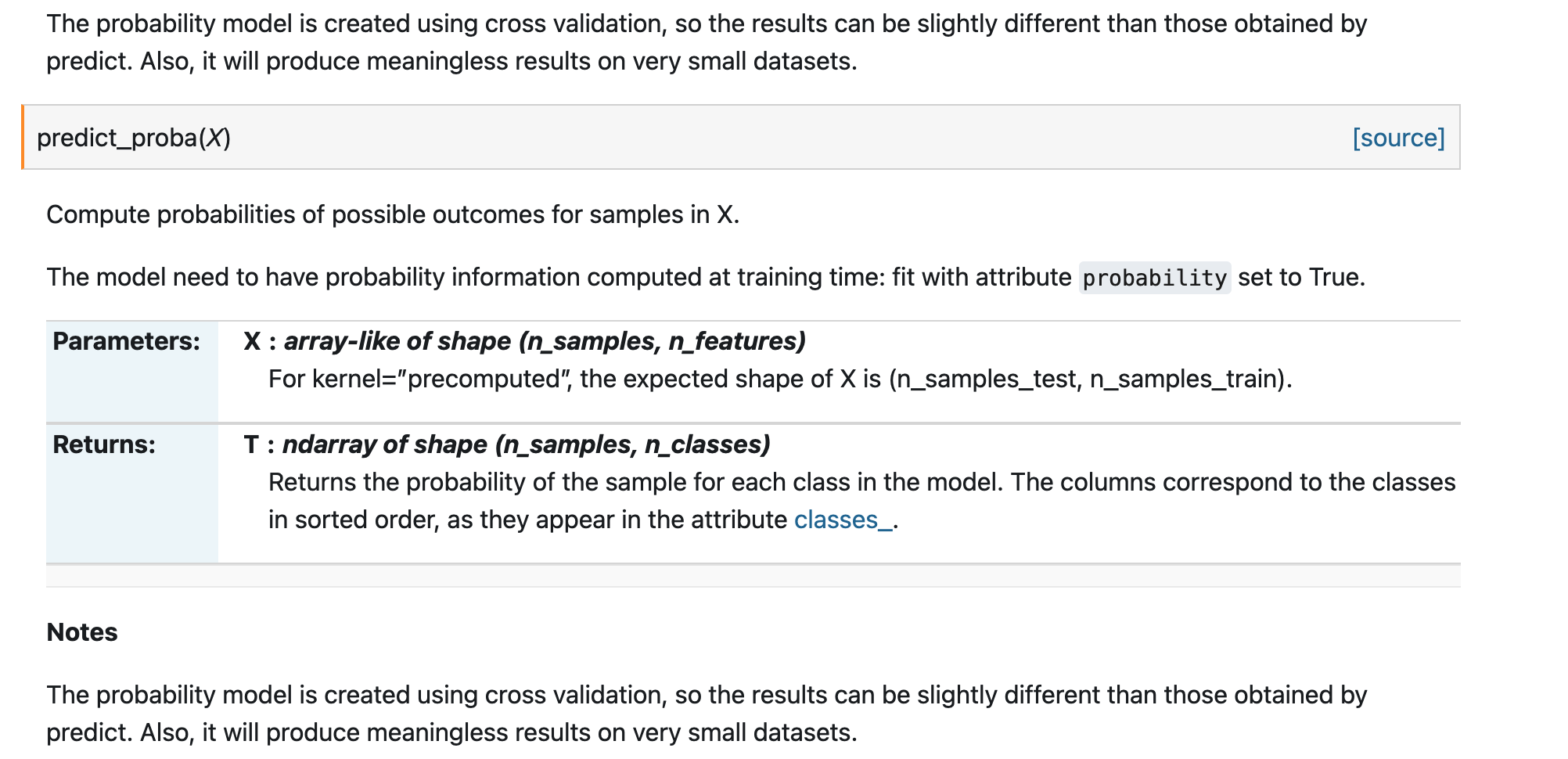• sklearn svm基本使用
千次阅读
2020-12-11 13:08:41

SVM基本使用

SVM在解决分类问题具有良好的效果，出名的软件包有libsvm(支持多种核函数),liblinear。此外python机器学习库scikit-learn也有svm相关算法，sklearn.svm.SVC和

sklearn.svm.LinearSVC分别由libsvm和liblinear发展而来。

推荐使用SVM的步骤为：

将原始数据转化为SVM算法软件或包所能识别的数据格式；

将数据标准化；(防止样本中不同特征数值大小相差较大影响分类器性能)

不知使用什么核函数，考虑使用RBF；

利用交叉验证网格搜索寻找最优参数(C, γ)；(交叉验证防止过拟合，网格搜索在指定范围内寻找最优参数)

使用最优参数来训练模型；

测试。

下面利用scikit-learn说明上述步骤：

1 importnumpy as np2 from sklearn.svm importSVC3 from sklearn.preprocessing importStandardScaler4 from sklearn.model_selection importGridSearchCV, train_test_split5

8 假设这是鸢尾花数据,csv数据格式为：9 0,5.1,3.5,1.4,0.210 0,5.5,3.6,1.3,0.511 1,2.5,3.4,1.0,0.512 1,2.8,3.2,1.1,0.213 每一行数据第一个数字(0,1...)是标签,也即数据的类别。14 '''

15 data = np.genfromtxt(filename, delimiter=',')16 x = data[:, 1:] #数据特征

17 y = data[:, 0].astype(int) #标签

18 scaler =StandardScaler()19 x_std = scaler.fit_transform(x) #标准化

20 #将数据划分为训练集和测试集，test_size=.3表示30%的测试集

21 x_train, x_test, y_train, y_test = train_test_split(x_std, y, test_size=.3)22 returnx_train, x_test, y_train, y_test23

24

25 defsvm_c(x_train, x_test, y_train, y_test):26 #rbf核函数，设置数据权重

27 svc = SVC(kernel='rbf', class_weight='balanced',)28 c_range = np.logspace(-5, 15, 11, base=2)29 gamma_range = np.logspace(-9, 3, 13, base=2)30 #网格搜索交叉验证的参数范围，cv=3,3折交叉

31 param_grid = [{'kernel': ['rbf'], 'C': c_range, 'gamma': gamma_range}]32 grid = GridSearchCV(svc, param_grid, cv=3, n_jobs=-1)33 #训练模型

34 clf =grid.fit(x_train, y_train)35 #计算测试集精度

36 score =grid.score(x_test, y_test)37 print('精度为%s' %score)38

39 if __name__ == '__main__':

其它内容

网格搜索小技巧

网格搜索法中寻找最优参数中为寻找最优参数，网格大小如果设置范围大且步长密集的话难免耗时，但是不这样的话又可能找到的参数不是很好，针对这解决方法是，先在大范围，大步长的粗糙网格内寻找参数。在找到的参数左右在设置精细步长找寻最优参数比如：

一开始寻找范围是 C = 2−5 , 2 −3 , . . . , 2 15 and γ = 2−15 , 2 −13 , . . . , 2 3 .由此找到的最优参数是(23 , 2 −5 )；

然后设置更小一点的步长，参数范围变为21 , 2 1.25 , . . . , 2 5 and γ = 2−7 , 2 −6.75 , . . . , 2−3 在这个参数范围再寻找最优参数。

这样既可以避免一开始就使用大范围，小步长而导致分类器进行过于多的计算而导致计算时间的增加。

线性核和RBF的选择

如果训练样本的特征数量过于巨大，也许就不需要通过RBF等非线性核函数将其映射到更高的维度空间上，利用非线性核函数也并不能提高分类器的性能。利用linear核函数也可以获得足够好的结果，此外，也只需寻找一个合适参数C，但是利用RBF核函数取得与线性核函数一样的效果的话需要寻找两个合适参数(C, γ)。

分三种情况讨论：

样本数量远小于特征数量：这种情况，利用情况利用linear核效果会高于RBF核。

样本数量和特征数量一样大：线性核合适，且速度也更快。liblinear更适合

样本数量远大于特征数量： 非线性核RBF等合适。

其它文章：

更多相关内容
• ## sklearnSVM函数

千次阅读 2019-11-20 21:29:01
sklearn SVM函数属性和参数 属性和参数

# 属性和参数

## 参数

• C: float参数 默认值为1.0
错误项的惩罚系数。C越大，即对分错样本的惩罚程度越大，因此在训练样本中准确率越高，但是泛化能力降低，也就是对测试数据的分类准确率降低。相反，减小C的话，容许训练样本中有一些误分类错误样本，泛化能力强。对于训练样本带有噪声的情况，一般采用后者，把训练样本集中错误分类的样本作为噪声。

• kernel: str参数 默认为‘rbf’
算法中采用的核函数类型，可选参数有：
‘linear’:线性核函数
‘poly’：多项式核函数
‘rbf’：径像核函数/高斯核
‘sigmod’:sigmod核函数
‘precomputed’:核矩阵

• degree: int型参数 默认为3
这个参数只对多项式核函数有用，是指多项式核函数的阶数n
如果给的核函数参数是其他核函数，则会自动忽略该参数。

• gamma: float参数 默认为auto
核函数系数，只对‘rbf’,‘poly’,‘sigmod’有效。
如果gamma为auto，代表其值为样本特征数的倒数，即1/n_features.

• coef0: float参数 默认为0.0
核函数中的独立项，只有对‘poly’和‘sigmod’核函数有用，是指其中的参数c

• probability： bool参数 默认为False
是否启用概率估计。 这必须在调用fit()之前启用，并且会fit()方法速度变慢。

• shrinking： bool参数 默认为True
是否采用启发式收缩方式

• tol: float参数 默认为1e^-3
svm停止训练的误差精度

• cache_size： float参数 默认为200
指定训练所需要的内存，以MB为单位，默认为200MB。

• class_weight： 字典类型或者‘balance’字符串。默认为None
给每个类别分别设置不同的惩罚参数C，如果没有给，则会给所有类别都给C=1，即前面参数指出的参数C.
如果给定参数‘balance’，则使用y的值自动调整与输入数据中的类频率成反比的权重。

• verbose： bool参数 默认为False
是否启用详细输出。 此设置利用libsvm中的每个进程运行时设置，如果启用，可能无法在多线程上下文中正常工作。一般情况都设为False，不用管它。

• max_iter： int参数 默认为-1
最大迭代次数，如果为-1，表示不限制

• random_state： int型参数 默认为None
伪随机数发生器的种子,在混洗数据时用于概率估计。

• fit() 方法：用于训练SVM，具体参数已经在定义SVC对象的时候给出了，这时候只需要给出数据集X和X对应的标签y即可。

• predict() 方法: 基于以上的训练，对预测样本T进行类别预测，因此只需要接收一个测试集T，该函数返回一个数组表示个测试样本的类别。

• 属性有哪些

• svc.n_support_：各类各有多少个支持向量

• svc.support_：各类的支持向量在训练样本中的索引

• svc.support_vectors_：各类所有的支持向量

# 示例

import matplotlib.pyplot as plt
import numpy as np
from sklearn import datasets, linear_model, model_selection, svm

X_train = iris.data
y_train = iris.target
X_train, X_test, y_train, y_test = model_selection.train_test_split(X_train, y_train, test_size=0.25, random_state=0, stratify=y_train)

cls = svm.SVC(kernel='linear')
cls.fit(X_train, y_train)
print('Coefficients:%s, intercept %s' % (cls.coef_, cls.intercept_))
print('Score: %.2f' % cls.score(X_test, y_test))


## poly + degree

fig = plt.figure()

degrees = range(1, 20)
train_scores = []
test_scores = []
for degree in degrees:
cls = svm.SVC(kernel='poly', degree=degree)
cls.fit(X_train, y_train)
train_scores.append(cls.score(X_train, y_train))
test_scores.append(cls.score(X_test, y_test))

ax.plot(degrees, train_scores, label="Training score ", marker='+')
ax.plot(degrees, test_scores, label=" Testing  score ", marker='o')
ax.set_title("SVC_poly_degree ")
ax.set_xlabel("p")
ax.set_ylabel("score")
ax.set_ylim(0, 1.05)
ax.legend(loc="best", framealpha=0.5)

gammas = range(1, 20)
train_scores = []
test_scores = []
for gamma in gammas:
cls = svm.SVC(kernel='poly', gamma=gamma, degree=3)
cls.fit(X_train, y_train)
train_scores.append(cls.score(X_train, y_train))
test_scores.append(cls.score(X_test, y_test))
ax.plot(gammas, train_scores, label="Training score ", marker='+')
ax.plot(gammas, test_scores, label=" Testing  score ", marker='o')
ax.set_title("SVC_poly_gamma ")
ax.set_xlabel(r"$\gamma$")
ax.set_ylabel("score")
ax.set_ylim(0, 1.05)
ax.legend(loc="best", framealpha=0.5)

rs = range(0, 20)
train_scores = []
test_scores = []
for r in rs:
cls = svm.SVC(kernel='poly', gamma=10, degree=3, coef0=r)
cls.fit(X_train, y_train)
train_scores.append(cls.score(X_train, y_train))
test_scores.append(cls.score(X_test, y_test))
ax.plot(rs, train_scores, label="Training score ", marker='+')
ax.plot(rs, test_scores, label=" Testing  score ", marker='o')
ax.set_title("SVC_poly_r ")
ax.set_xlabel(r"r")
ax.set_ylabel("score")
ax.set_ylim(0, 1.05)
ax.legend(loc="best", framealpha=0.5)
plt.show()## rbf + gamma

gammas = range(1, 20)
train_scores = []
test_scores = []
for gamma in gammas:
cls = svm.SVC(kernel='rbf', gamma=gamma)
cls.fit(X_train, y_train)
train_scores.append(cls.score(X_train, y_train))
test_scores.append(cls.score(X_test, y_test))
fig = plt.figure()
ax.plot(gammas, train_scores, label="Training score ", marker='+')
ax.plot(gammas, test_scores, label=" Testing  score ", marker='o')
ax.set_title("SVC_rbf")
ax.set_xlabel(r"$\gamma$")
ax.set_ylabel("score")
ax.set_ylim(0, 1.05)
ax.legend(loc="best", framealpha=0.5)
plt.show()## sigmoid + gamma + coef

fig = plt.figure()

gammas = np.logspace(-2, 1)
train_scores = []
test_scores = []

for gamma in gammas:
cls = svm.SVC(kernel='sigmoid', gamma=gamma, coef0=0)
cls.fit(X_train, y_train)
train_scores.append(cls.score(X_train, y_train))
test_scores.append(cls.score(X_test, y_test))
ax.plot(gammas, train_scores, label="Training score ", marker='+')
ax.plot(gammas, test_scores, label=" Testing  score ", marker='o')
ax.set_title("SVC_sigmoid_gamma ")
ax.set_xscale("log")
ax.set_xlabel(r"$\gamma$")
ax.set_ylabel("score")
ax.set_ylim(0, 1.05)
ax.legend(loc="best", framealpha=0.5)

rs = np.linspace(0, 5)
train_scores = []
test_scores = []

for r in rs:
cls = svm.SVC(kernel='sigmoid', coef0=r, gamma=0.01)
cls.fit(X_train, y_train)
train_scores.append(cls.score(X_train, y_train))
test_scores.append(cls.score(X_test, y_test))
ax.plot(rs, train_scores, label="Training score ", marker='+')
ax.plot(rs, test_scores, label=" Testing  score ", marker='o')
ax.set_title("SVC_sigmoid_r ")
ax.set_xlabel(r"r")
ax.set_ylabel("score")
ax.set_ylim(0, 1.05)
ax.legend(loc="best", framealpha=0.5)
plt.show()展开全文机器学习
• @python,sklearn,svm,遥感数据分类,代码实例 python_sklearn_svm遥感数据分类代码实例 （1）svm原理简述 支持向量机（Support Vector Machine，即SVM）是包括分类（Classification）、回归(Regression)和异常检测...
• 1.SVM简介 支持向量机（Support Vector Machine, SVM）是一类按监督学习（supervised learning）方式对数据进行二元分类（binary classification）的广义线性分类器（generalized linear classifier），其决策边界是...

## 1.SVM简介

支持向量机（Support Vector Machine, SVM）是一类按监督学习（supervised learning）方式对数据进行二元分类（binary classification）的广义线性分类器（generalized linear classifier），其决策边界是对学习样本求解的最大边距超平面（maximum-margin hyperplane）。
SVM的基本思想是：找到集合边缘上的若干数据（称为支持向量（Support Vector）），用这些点找出一个平面（称为决策面），使得支持向量到该平面的距离最大。

## 2.SVM参数意义

在sklearn中，封装好各种机器学习的库，其中就包含SVM算法，其调用如下：

import sklearn.svm as svm
model = svm.SVC(C=1.0,
kernel='rbf',
degree=3,
gamma='auto',
coef0=0.0,
shrinking=True,
probability=False,
tol=0.001,
cache_size=200, c
lass_weight=None,
verbose=False,
max_iter=-1,
decision_function_shape=None,
random_state=None)


参数说明：
C：SVC的惩罚参数，默认值是1.0；C越大，对误分类的惩罚增大，趋向于对训练集完全分对的情况，这样对训练集测试时准确率很高，但泛化能力弱C值小，对误分类的惩罚减小，允许容错，将他们当成噪声点，泛化能力较强

关于松弛变量，我这边要解释下：
在大多数情况下，数据并不是完美的线性可分数据，可能会存在少量的点出现在分类超平面的另外一侧。我们希望尽量保证将这些点进行正确分类，同时又保证分类面与两类样本点有足够大的几何间隔。在这种情况下，我们为每一个样本点加上一个松弛变量，允许有小的误差存在。在加入松弛变量后，我们还要在目标函数中加入相应的惩罚参数C，对这个松弛变量起到一个监督克制的作用。两者的关系，有点类似道家的阴阳制衡的关系，此消彼长。

kernel ：核函数，默认是rbf，可以是‘linear’, ‘poly’, ‘rbf’, ‘sigmoid’, ‘precomputed’
linear：线性分类器（C越大分类效果越好，但有可能会过拟合（default C=1））
poly：多项式分类器
rbf：高斯模型分类器（gamma值越小，分类界面越连续；gamma值越大，分类界面越“散”，分类效果越好，但有可能会过拟合。）
sigmoid：sigmoid核函数
具体可以参考：https://zhuanlan.zhihu.com/p/157722218
degree ：多项式poly函数的维度，默认是3，选择其他核函数时会被忽略。
gamma ： ‘rbf’,‘poly’ 和‘sigmoid’的核函数参数。默认是’auto’。 如果gamma是’auto’，那么实际系数是1 / n_features。
coef0 ：核函数中的独立项。 它只在’poly’和’sigmoid’中很重要。
probability ：是否启用概率估计。 必须在调用fit之前启用它，并且会减慢该方法的速度。默认为False
shrinking ：是否采用shrinking heuristic方法(收缩启发式)，默认为true
tol ：停止训练的误差值大小，默认为1e-3
cache_size ：核函数cache缓存大小，默认为200
class_weight ：类别的权重，字典形式传递。设置第几类的参数C为weight*C(C-SVC中的C)
verbose ：允许冗余输出
max_iter ：最大迭代次数。-1为无限制。
decision_function_shape ：‘ovo’, ‘ovr’ or None, default=ovr
关于‘ovo’, ‘ovr’的解释：
一对多法（one-versus-rest,简称OVR SVMs）：训练时依次把某个类别的样本归为一类,其他剩余的样本归为另一类，这样k个类别的样本就构造出了k个SVM。分类时将未知样本分类为具有最大分类函数值的那类。
一对一法（one-versus-one,简称OVO SVMs或者pairwise）：其做法是在任意两类样本之间设计一个SVM，因此k个类别的样本就需要设计k(k-1)/2个SVM。当对一个未知样本进行分类时，最后得票最多的类别即为该未知样本的类别。
详细讲解，可以参考这篇博客：https://blog.csdn.net/xfchen2/article/details/79621396
random_state ：数据洗牌时的种子值，int值,default=None
在随机数据混洗时使用的伪随机数生成器的种子。 如果是int，则random_state是随机数生成器使用的种子; 如果是RandomState实例，则random_state是随机数生成器; 如果为None，则随机数生成器是np.random使用的RandomState实例。
个人认为最重要的参数有：C、kernel、degree、gamma、coef0、decision_function_shape。

## 3.sklearn中SVM接口调用

# 建立模型
model = svm.SVC(C=10, kernel='linear')

# 训练模型,x为训练集， y为标签
model.fit(x, y)

# 预测,test为测试集
pre_y = model.predict(test)

# 预测结果数据
n_Support_vector = clf.n_support_  # 支持向量个数
sv_idx = clf.support_  # 支持向量索引
Support_vector = clf.support_vectors_   # 支持向量
w = clf.coef_  # 权重矩阵W
b = clf.intercept_  # 偏置矩阵b


## 4.代码实现

运行环境：pycharm、scikit-learn 0.23.2

import numpy as np
import joblib
from sklearn import svm
import matplotlib.pyplot as plt
x = [[1, 2], [4, 5], [18, 9], [12, 6], [2, 3], [13, 18]]
x = np.array(x)
y = [1, 1, 0, 0, 1, 0]
y = np.array(y)
# 训练模型
model = svm.SVC(C=10, kernel='linear')
model.fit(x, y)

# 预测
a = [[8, 6]]
a_pre = model.predict(a)
print("a_pre:", a_pre)
# 对应的支持向量
Support_vector = model.support_vectors_
print("Support_vector:", Support_vector)
# 线性分类对应的参数
w = model.coef_
print("w:", w)
b = model.intercept_
print("b:", b)
# 训练集散点图
plt.scatter(x[:, 0], x[:, 1])

if w[0, 1] != 0:
xx = np.arange(0, 20, 0.1)
# 最佳分类线
yy = -w[0, 0]/w[0, 1] * xx - b/w[0, 1]
plt.scatter(xx, yy, s=4)
# 支持向量
b1 = Support_vector[0, 1] + w[0, 0]/w[0, 1] * Support_vector[0, 0]
b2 = Support_vector[1, 1] + w[0, 0]/w[0, 1] * Support_vector[1, 0]
yy1 = -w[0, 0] / w[0, 1] * xx + b1
plt.scatter(xx, yy1, s=4)
yy2 = -w[0, 0] / w[0, 1] * xx + b2
plt.scatter(xx, yy2, s=4)
else:
xx = np.ones(100) * (-b) / w[0, 0]
yy = np.arange(0, 10, 0.1)
plt.scatter(xx, yy)
plt.show()

展开全文机器学习
• 查阅sklearn官方文档可知，predict_log_proba(X)这个函数计算X中的样本可能结果的概率。但是该概率的计算使用交叉验证创建概率模型，因此结果可以略有不同于预测获得的模型。此外，它将在非常小的数据集上产生毫无...

查阅sklearn官方文档可知，predict_log_proba(X)这个函数计算X中的样本可能结果的概率。但是该概率的计算使用交叉验证创建概率模型，因此结果可以略有不同于预测获得的模型。此外，它将在非常小的数据集上产生毫无意义的结果。展开全文python
• 在调用sklearnSVM时，如果设置verbose=True,模型训练结束后会显示一些训练过程的说明信息，如下(以下是OCSVM的返回结果)： * optimization finished, # iter = 32 obj = 192.154077, rho = 19.244379 nSV = 26, ...
• sklearn 做多分类时，svm.predict_proba每类概率具体是如何算法机器学习 python
• ## sklearnSVM使用

万次阅读 多人点赞 2018-01-17 17:49:12python 机器学习
• 本次实验并不要求实现SVM，只需要调通各种库（例如MATLAB、sklearn等等）里已经集成好的函数即可，所以一开始我觉得很简单（事实上也确实很简单），但是在这个过程中因为我写代码不够规范，而出现了一些意想不到的...python 机器学习
• 机器学习分类器——案例（opencv sklearn svm ann） 训练多分类器，提取细胞核特征，进行预测。opencv 目标检测 机器学习 分类
• from sklearn.svm import SVC clf = SVC(kernel='rbf')#调参 clf.fit(X, y)#训练 print(clf.fit(X, y))#输出参数设置 p = 1#正确分类的个数 for i in range(len(test_dataset)):#循环检测测试数据分类成功的个数 ...二分类
• 文件下载地址： ...SVM 线性核函数 """ 线性核函数的 SVM分类器 """ import numpy as np import sklearn.naive_bayes as nb ...import sklearn.svm as svm import sklearn.model_selection as ms impo
• 正在学习sklearn , 实验室项目需要实现一些文本的分类的功能。 sklearn提供了许多机器学习方面使用的到的写好的工具。其中也包括分类器。sklearn在这里不作介绍。有官网，有博客，也正在学习中最开始是参照着这片...机器学习
• 在前面的一篇文章我们给出了使用Sklearn 中的SVM进行分类时如何使用留一法来进行分类。 python基于sklearnSVM和留一法（LOOCV）进行二分类 如何在使用留一法时绘制ROC曲线和AUC 什么是ROC曲线和？这个可以参考如下...python 算法
• #导入，处理数据集import pandas as pd ...from sklearn import svm from sklearn.model_selection import GridSearchCV from sklearn.model_selection import train_test_splitdataset1=pd.read_excel("http机器学习
• dual_coef_返回的内容为 SVM对偶问题的所求得的支持向量对应的 拉格朗日系数与标签的乘机，即alpha_i* y_i ...from sklearn.svm import SVC from sklearn import datasets from sklearn.model_selection import tr..
• from sklearn import svm import matplotlib.pyplot as plt import numpy as np from sklearn.datasets import load_iris import numpy as np import random import numpy.random as rand rand.seed(10) # 读取...
• Sklearn.svm.LinearSVC(penalty=’l2’, loss=’squared_hinge’, dual=True, tol=0.0001, C=1.0, multi_class=’ovr’,fit_intercept=True, intercept_scaling=1, class_weight=None, verbose=0, random_state=None...
• 由于SVM是需要不断的寻找可以最优区分开数据之间的超平面直到计算出的结果收敛为止，但由于数据的变化，例如区分高矮的准则是，超过180cm就是高，否则就不是的这种，当你给出身高的数据时，数据间具有明显的线性关系...机器学习 python
• ## sklearnsvm.LinearSVC的参数说明

万次阅读 多人点赞 2018-07-27 14:29:11
Sklearn.svm.LinearSVC参数说明 与参数kernel ='linear'的SVC类似，但是以liblinear而不是libsvm的形式实现，因此它在惩罚和损失函数的选择方面具有更大的灵活性，并且应该更好地扩展到大量样本。 此类支持密集和...
• https://www.cnblogs.com/huanping/p/9330849.html
• sklean SVM（支持向量机）模型使用RandomSearchCV获取最优参数及可视化 支持向量机（support vector machines, SVM）是一种二分类模型，它的基本模型是定义在特征空间上的间隔最大的线性分类器，间隔最大使它有...可视化 机器学习 python 支持向量机 数据挖掘
• 利用 sklearn SVM 分类器对 IRIS 数据集分类 支持向量机（SVM）是一种最大化分类间隔的线性分类器（如果不考虑核函数）。通过使用核函数可以用于非线性分类。SVM 是一种判别模型，既适用于分类也适用于回归问题，...python
• 于是这篇来总结一下sklearnsvm的参数说明以及调参经验。方便以后查询和回忆。常用核函数1.linear核函数:K(xi,xj)=xTixjK(xi,xj)=xiTxj2.polynomial核函数:K(xi,xj)=(γxTixj+r)d,d>1K(xi,xj)=(γxiTxj+r)d,d&g....
• 文章目录一、SVM算法二、算法实现1.引包2.加载数据3.修改数据4.定义函数5.线性处理6.非线性处理7.核函数处理8.高斯核函数处理三、总结四、参考 一、SVM算法 将实例的特征向量（以二维为例）映射为空间中的一些点，如...支持向量机 算法
• 对于机器学习的一些模型，跑完之后，如果下一次测试又需要重新跑一遍模型是一件很繁琐的事，这时候我们就需要保存模型，再加载调用。 楼主发现有这些保存模型的方法，网上有很多错误的例子，所以给...f=open('svm...model save
• sklearn中有关SVM预测可视化的借鉴。 目前，仅实现了二维（特征）的可视化。支持向量机 机器学习
• 利用sklearnsvm预测趋势数据准备模型训练和预测 数据准备 def get_data(code,start,end,window_len=20): ts_pro=ts.pro_api('xx') df=ts_pro.daily(ts_code=code,start_date=start,end_date=end,fields='ts_code...python 支持向量机
• 我正在尝试构建一个垃圾邮件分类器，我从互联网上收集了多个数据集(例如，垃圾邮件/垃圾邮件的SpamAssassin数据库)并构建了以下内容：import osimport numpyfrom pandas import DataFramefrom sklearn.feature_...
• from sklearn.svm import LinearSVC from sklearn.svm import SVC 性感官方，在线文档 性感官方，在线文档 参数 #线性SVC penalty惩罚项l1 or l2，默认l2. loss损失函数的选择，hinge or squared_hinge。 dual是否...机器学习...